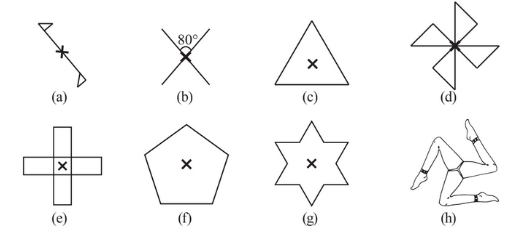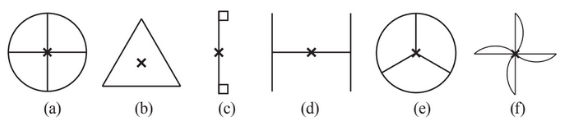## Filters

Sort by :
Clear All
Q

2. Give the order of rotational symmetry for each figure:(a) The given figure has rotational symmetry about  so it has ordered as 2.   (b) The given figure has rotational symmetry about  so it has ordered as 2.   (c) The given figure has rotational symmetry about  so it has ordered as 3.   (d) The given figure has rotational symmetry about  so it has ordered as 4.   (e) The given figure has rotational symmetry about  so it has ordered as 4.   (f) The...

1. Which of the following figures have rotational symmetry of order more than 1:Among the above-given shapes, (a),(b), (d),(e) and (f) have more than one rotational symmetry. This is because, in these figures, a complete turn, more than 1 number of times, an object look exactly the same.
Exams
Articles
Questions